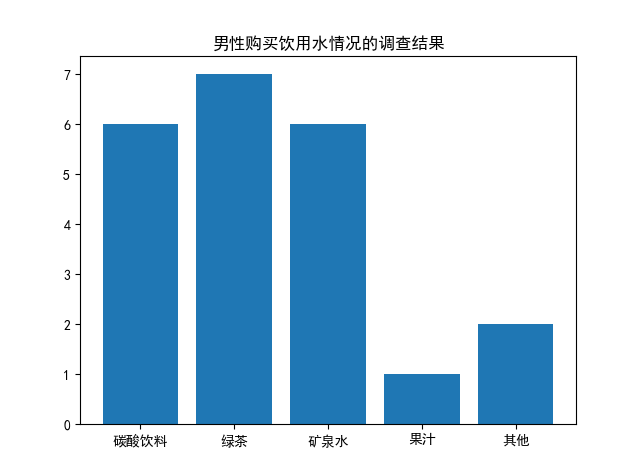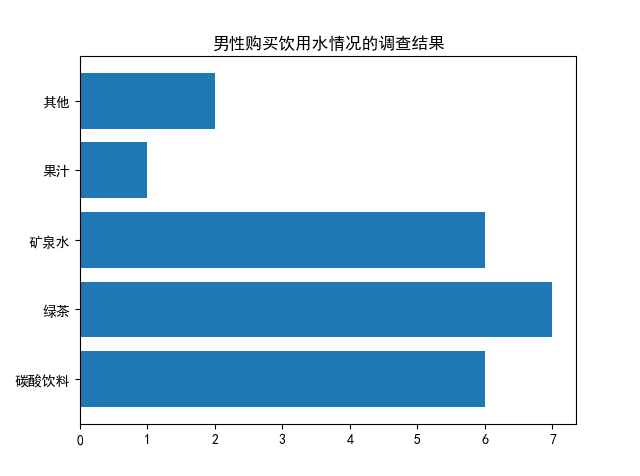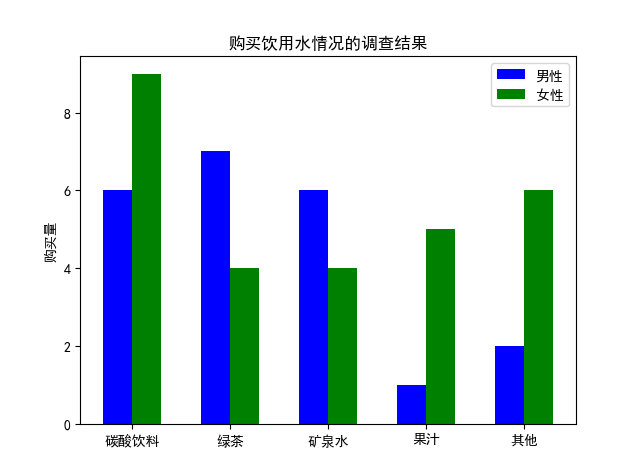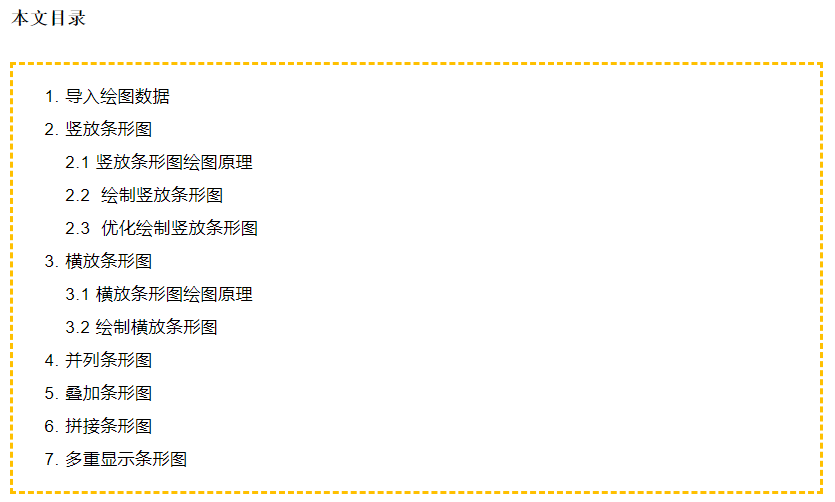• importmatplotlib.pyplotasplt importnumpyasnp importjson importmatplotlib.font_managerasfont_manager importpandasaspd #显示matplotlib生成的图形 %matplotlibinline ...#[40,45],...
import matplotlib.pyplot as plt
import numpy as np
import json
import matplotlib.font_manager as font_manager
import pandas as pd

#显示matplotlib生成的图形
%matplotlib inline

#print(df)

#[40,45],[45,50],[50,55],[55,60]
a_d=0
b_d=0
c_d=0
d_d=0
e_d=0
for weight_data in df['weight']:
weight_data = float(weight_data.replace("kg", ""))
if (40 <= weight_data <45):
a_d +=1
elif (45 <= weight_data <50):
b_d +=1
elif (50 <= weight_data <55):
c_d +=1
elif (55 <= weight_data <60):
d_d +=1
else: e_d +=1

s["40-45"]=a_d
s["45-50"]=b_d
s["50-55"]=c_d
s["55-60"]=d_d
s["other"]=e_d

zone_list = s.index
count_list = s.values

# 设置显示中文
plt.rcParams['font.sans-serif'] = ['SimHei'] # 指定默认字体

plt.figure(figsize=(20,15))

plt.bar(range(len(count_list)), count_list,color='r',tick_label=zone_list,facecolor='#9999ff',edgecolor='white')

# 这里是调节横坐标的倾斜度，rotation是度数，以及设置刻度字体大小
plt.xticks(rotation=45,fontsize=20)
plt.yticks(fontsize=20)

plt.legend()
plt.title('''table''',fontsize = 24)
plt.savefig('/home/aistudio/work/bar_result04.jpg')
plt.show()
展开全文• 主要介绍了python条形图(柱状图)实例，具有很好的参考价值，希望对大家有所帮助。一起跟随小编过来看看吧
• ## python 画条形图（柱状图）

万次阅读 多人点赞 2019-08-24 00:06:35
竖放条形图bar(x, height, [width], **kwargs)2. 画横放条形图bar(x, width, [height], **kwargs)3. 并列条形图 条形图（bar chart），也称为柱状图，是一种以长方形的长度为变量的统计图表，长方形的长度与它所...


文章目录
1. 竖放条形图bar(x, height, [width], **kwargs)

2. 横放条形图bar(x, width, [height], **kwargs)

3. 并列条形图

条形图（bar chart），也称为柱状图，是一种以长方形的长度为变量的统计图表，长方形的长度与它所对应的变量数值呈一定比例。

1. 竖放条形图
画条形图要用到 pyplot 中的 bar 函数，该函数的基本语法为：

bar(x, height, [width], **kwargs)

x数组，每个条形的横坐标height个数或一个数组，条形的高度[width]可选参数，一个数或一个数组，条形的宽度，默认为 0.8**kwargs不定长的关键字参数，用字典形式设置条形图的其他属性
**kwargs 中常设置的参数包括图形标签 label，颜色标签 color，不透明度 alpha 等。
假设某项针对男女大学生购买饮用水爱好的调查结果如下表：
男女碳酸饮料69绿茶74矿泉水64其他26果汁15总计2228
画出男生饮用水情况的直方图，代码如下：
import matplotlib.pyplot as plt

# 这两行代码解决 plt 中文显示的问题
plt.rcParams['font.sans-serif'] = ['SimHei']
plt.rcParams['axes.unicode_minus'] = False

waters = ('碳酸饮料', '绿茶', '矿泉水', '果汁', '其他')
buy_number = [6, 7, 6, 1, 2]

plt.title('男性购买饮用水情况的调查结果')

plt.show()

图形：2. 横放条形图
若要生成横的条形图，则可以使用 barh 函数，其语法与 bar 函数非常类似。

bar(x, width, [height], **kwargs)

y数组，每个条形的纵坐标width一个数或一个数组，条形的宽度[height]可选参数，一个数或一个数组，条形的高度，默认为 0.8**kwargs不定长的关键字参数，用字典形式设置条形图的其他属性
代码：
import matplotlib.pyplot as plt

# 这两行代码解决 plt 中文显示的问题
plt.rcParams['font.sans-serif'] = ['SimHei']
plt.rcParams['axes.unicode_minus'] = False

waters = ('碳酸饮料', '绿茶', '矿泉水', '果汁', '其他')
buy_number = [6, 7, 6, 1, 2]

plt.title('男性购买饮用水情况的调查结果')

plt.show()3. 并列条形图
若要将男生与女生的调查情况画出两个条形图一块显示，则可以使用 bar 或 barh 函数两次，并调整 bar 或 barh 函数的条形图位置坐标以及相应刻度，使得两组条形图能够并排显示。
import matplotlib.pyplot as plt
import numpy as np

# 这两行代码解决 plt 中文显示的问题
plt.rcParams['font.sans-serif'] = ['SimHei']
plt.rcParams['axes.unicode_minus'] = False

# 输入统计数据
waters = ('碳酸饮料', '绿茶', '矿泉水', '果汁', '其他')
buy_number_male = [6, 7, 6, 1, 2]
buy_number_female = [9, 4, 4, 5, 6]

bar_width = 0.3  # 条形宽度
index_male = np.arange(len(waters))  # 男生条形图的横坐标
index_female = index_male + bar_width  # 女生条形图的横坐标

# 使用两次 bar 函数画出两组条形图

plt.legend()  # 显示图例
plt.xticks(index_male + bar_width/2, waters)  # 让横坐标轴刻度显示 waters 里的饮用水， index_male + bar_width/2 为横坐标轴刻度的位置
plt.ylabel('购买量')  # 纵坐标轴标题
plt.title('购买饮用水情况的调查结果')  # 图形标题

plt.show()

显示：展开全文柱状图 bar
• 1.首先要绘制一个简单的条形图import numpy as npimport matplotlib.pyplot as pltfrom matplotlib import mlabfrom matplotlib import rcParamsfig1 = plt.figure(2)rects =plt.bar(left = (0.2,1),height = (1,0.5...
1.首先要绘制一个简单的条形图
import numpy as np
import matplotlib.pyplot as plt
from matplotlib import mlab
from matplotlib import rcParams
fig1 = plt.figure(2)
rects =plt.bar(left = (0.2,1),height = (1,0.5),width = 0.2,align="center",yerr=0.000001)
plt.title('Pe')
plt.show()1.1上面中rects=plt.bar(left=(0.2,1),height=(1,0.5),width=0.2,align=”center”,yerr=0.000001)这句代码是最重要的，其中left表示直方图的开始的位置（也就是最左边的地方），height是指直方图的高度，当直方图太粗时，可以通过width来定义直方图的宽度，注意多个直方图要用元组，yerr这个参数是防止直方图触顶。
2.增加直方图脚注
import numpy as np
import matplotlib.pyplot as plt
from matplotlib import mlab
from matplotlib import rcParams
fig1 = plt.figure(2)
rects =plt.bar(left = (0.2,1),height = (1,0.5),width = 0.2,align="center",yerr=0.000001)
plt.title('Pe')
plt.xticks((0.2,1),('frst','second'))
plt.show()3.条形图上显示具体的数字（自动编号）
import numpy as np
import matplotlib.pyplot as plt
from matplotlib import mlab
from matplotlib import rcParams
fig1 = plt.figure(2)
rects =plt.bar(left = (0.2,1),height = (1,0.5),width = 0.2,align="center",yerr=0.000001)
plt.title('Pe')
def autolabel(rects):
for rect in rects:
height = rect.get_height()
plt.text(rect.get_x()+rect.get_width()/2., 1.03*height, '%s' % float(height))
autolabel(rects)
plt.xticks((0.2,1),('frst','second'))
plt.show()4.改变颜色
import numpy as np
import matplotlib.pyplot as plt
from matplotlib import mlab
from matplotlib import rcParams
fig1 = plt.figure(2)
rects =plt.bar(left = (0.2,1),height = (1,0.5),color=('r','g'),width = 0.2,align="center",yerr=0.000001)
plt.title('Pe')
def autolabel(rects):
for rect in rects:
height = rect.get_height()
plt.text(rect.get_x()+rect.get_width()/2., 1.03*height, '%s' % float(height))
autolabel(rects)
plt.xticks((0.2,1),('frst','second'))
plt.show()5.添加图注
import numpy as np
import matplotlib.pyplot as plt
from matplotlib import mlab
from matplotlib import rcParams
fig1 = plt.figure(2)
rects1 =plt.bar(left = (0.2),height = (0.5),color=('g'),label=(('no1')),width = 0.2,align="center",yerr=0.000001)
rects2 =plt.bar(left = (1),height = (1),color=('r'),label=(('no2')),width = 0.2,align="center",yerr=0.000001)
plt.legend()
plt.xticks((0.2,1),('frst','second'))
plt.title('Pe')
def autolabel(rects):
for rect in rects:
height = rect.get_height()
plt.text(rect.get_x()+rect.get_width()/2., 1.03*height, '%s' % float(height))
autolabel(rects1)
autolabel(rects2)
plt.show()6大家根据自己的需要自己来绘制自己的条形图
下面回答网友提问，如何画在条形图上垂直显示数据：
下面这个函数是用来垂直显示的，其中设置角度就可以以任意方式来显示。
def autolabel(rects,Num=1.12,rotation1=90,NN=1):
for rect in rects:
height = rect.get_height()
plt.text(rect.get_x()-0.04+rect.get_width()/2., Num*height, '%s' % float(height*NN),rotation=rotation1)
调用方式如下
rects1 =plt.bar(left = (0.05),height = (Pe_FH),color=('b'),label=('FHMM'),width = 0.1,align="center",yerr=0.000001);
autolabel(rects1,1.09);
下面是效果图总结
以上就是本文关于python绘制条形图方法代码详解的全部内容，希望对大家有所帮助。感兴趣的朋友可以继续参考本站：
如有不足之处，欢迎留言指出。感谢朋友们对本站的支持！
展开全文• 让你彻底掌握在python中绘制条形图
条形图（bar chart）也称为柱状图，是一种以长方形的长度为变量的统计图表，长方形的长度与它所对应的数值呈一定比例。一、导入绘图数据首先导入绘图所需的数据。
import os
import pandas as pd

os.chdir(r'F:\公众号\6.学习python')   #设置成存放数据文件夹路径
date = pd.read_csv("股票数据.csv", encoding = 'GBK')    #读取数据

注：如需文中数据进行练习，可到公众号中回复“股票数据波动”即可免费获取。展示前几行数据如下：二、竖放条形图1 竖放条形图绘图原理
Python中绘制竖放条形图需用matplotlib.pyplot中的bar函数，该函数的基本语法为：
bar(x, height, [width], [bottom],  **kwargs)

参数说明：    x：数组，条形图对应的横坐标。    height：一个数或数组，条形图的纵坐标（高度）。    [width]：一个数或数组，条形的宽度，默认值0.8，为可选参数。    [bottom]：一个数或数组，条形的起始高度，默认值0，为可选参数。    **kwargs：不定长关键字参数，用字典形式设置条形图的其它参数。
2 绘制竖放条形图
以日期为横坐标，收盘价为纵坐标绘制竖放条形图，具体语句如下：
import matplotlib.pyplot as plt           #导入库

date = date.set_index('日期')             #把日期列设为索引
date.index = pd.to_datetime(date.index)   #把索引转为时间格式
plt.bar(date.index, date['收盘价'])       #以日期为横轴，收盘价为纵轴绘制条形图

得到结果如下：从以上结果可以发现，由于数据较多，条形图密密麻麻，看不出具体趋势。故在绘图之前，最好先进行统计汇总。

3 优化显示竖放条形图
以时间为横轴，每年收盘价均值为纵轴绘制竖放条形图，并添加标题和轴标签等，具体语句如下：
result = date[['收盘价']].groupby(date.index.year).mean() #计算每年收盘价均值

plt.rcParams['font.sans-serif'] = ['SimHei']  # 解决中文显示问题
plt.rcParams['axes.unicode_minus'] = False   # 解决中文显示问题

plt.bar(result.index, result['收盘价'], 0.6, label='close', color='#87CEFA')
plt.legend()                                 #显示图例
plt.xlabel('时间')                           #x轴标签
plt.ylabel('收盘价')                         #y轴标签
plt.title('股票收盘价')                      #标题

得到结果如下：三、横放条形图1 横放条形图绘图原理
Python中绘制横向条形图需用matplotlib.pyplot中的barh函数，该函数和bar函数类似，它的基本语法为：
barh(y, width, [height],  **kwargs)

参数说明：    y：一个数或数组，条形图对应的纵坐标。    width：一个数或数组，条形的宽度。    [height]：一个数或数组，条形的高度，默认值0.8，为可选参数。    **kwargs：不定长关键字参数，用字典形式设置条形图的其它参数。
2 绘制横放条形图
以时间为纵轴，收盘价为高度绘制横放条形图，具体语句如下：
result = date[['收盘价']].groupby(date.index.year).mean()  #计算每年收盘价均值
plt.barh(result.index, result['收盘价'])                   #以时间为纵轴，收盘价为高度绘制横向条形图

得到结果如下：四、并列条形图有时在绘制条形图时需对比显示某些信息，比如想同时观察股票最高价和最低价的变化趋势，可采用并列条形图，具体语句如下：
result = date.groupby(date.index.year).agg(high=('最高价','mean'), low=('最低价','mean')) #分别计算每年股票最高价、最低价均值
plt.bar(result.index, result['high'], width=0.2, color='r', label='high')                #绘制每年股票最高价均值的条形图，颜色设置为红色
plt.bar(result.index+0.2, result['low'], width=0.2, color ='b', label='low')             #绘制每年股票最低价均值的条形图，颜色设置为蓝色
plt.xticks(result.index+0.2, result.index)                                               # 让横坐标轴刻度显示时间，result.index+0.2为横坐标轴刻度的位置
plt.ylim(5, 35)                                                                          #设置y轴的显示范围
plt.title('股票最高价最低价')                                                            #设置标题
plt.legend()                                                                             #显示图例

得到结果如下：五、叠加条形图有时一个变量的数值恒小于另一个变量，这时可以把两个条形图绘制到一个条形图中，用不同的颜色显示这两个条形图即可。比如股票价格的最小值恒小于最大值，可以把这两个数组绘制在同一个条形图中，具体语句如下：
result = date.groupby(date.index.year).agg(high=('最高价','mean'), low=('最低价','mean')) #分别计算每年股票最高价、最低价均值
plt.bar(result.index, result['high'], width=0.2, color='r', alpha=0.5, label='high')      #绘制股票最高价条形图，颜色为红色
plt.bar(result.index, result['low'], width=0.2, color='b', alpha=0.5, label='low')        #绘制股票最低价条形图，颜色为蓝色
plt.title('叠加股票最高价最低价条形图')                                                   #设置标题
plt.legend()                                                                              #显示图例

得到结果如下：六、拼接条形图有时需要把两组数值绘制在同一个条形图中，以股票最高价和最低价为示例，绘制拼接条形图，具体语句如下：
result = date.groupby(date.index.year).agg(high=('最高价','mean'), low=('最低价','mean')) #分别计算每年股票最高价、最低价均值
plt.bar(result.index, result['high'], width=0.2, color='r', label='high')                 #绘制股票最高价条形图
plt.bar(result.index, result['low'], bottom=result['high'], width=0.2, color='b', label='low') #以股票最高价为起点绘制股票最低价条形图
plt.title('股票最高价最低价拼接条形图')                                                  #设置标题
plt.legend()        #显示图例

得到结果：七、多重显示条形图有时可以把一组数值看成高度，另一组数值代表颜色绘制多重显示条形图。以股票收盘价和换手率为例，绘制多重显示条形图，具体语句如下：
import matplotlib.cm as cm  #导入库

result = date.groupby(date.index.year).agg(avgl=('收盘价', 'mean'), avg2=('换手率', 'mean'))  #按年统计股票收盘价和换手率的均值
plt.bar(result.index, result['avgl'], color=cm.ScalarMappable().to_rgba(result['avg2']))      #以收盘价均值为高度，换手率均值为颜色绘制条形图
sm = plt.cm.ScalarMappable(norm=plt.Normalize(vmin=result['avg2'].min(), vmax=result['avg2'].max()))
plt.colorbar(sm)  #根据换手率均值的大小显示颜色
plt.title('用收盘价和换手率绘制多重显示条形图')  #设置标题
plt.show()

得到结果如下：至此，在Python中绘制条形图已全部讲解完毕，感兴趣的同学可以自己实现一遍。
你可能感兴趣: 用Python绘制皮卡丘 用Python绘制词云图 Python人脸识别—我的眼里只有你 Python画好看的星空图（唯美的背景） 用Python中的py2neo库操作neo4j，搭建关联图谱 Python浪漫表白源码合集（爱心、玫瑰花、照片墙、星空下的告白）长按(扫一扫)识别上方二维码学习更多Python和建模知识，让你的学习和工作更出彩。

展开全文数据可视化
• 条形图是一种用来描绘已汇总的分类型数据的频数分布、相对频数分布或百分数频数分布。（百分数频数就是相对频数乘以100%） 绘制条形图的主要方法： 方法1：通过pandas包中的Series对象或DataFrame对象调用plot()、...数据分析
• ## 用python画条形图

千次阅读 2019-04-09 16:01:19
在做毕设的过程中有些数据用表格来展现，会很难看出数据之间的差别，凸显不出数据的特点，所以想制作一个条形图，这里特地记录下，已备以后用到，这两张图对我这种小菜鸟来说可是花了一天的时间啊。。。。 import ...matplotlib
• 本文实例为大家分享了python读取mysql数据绘制条形图的具体代码，供大家参考，具体内容如下 Mysql 脚本示例： create table demo( id int ,product varchar(50) ,price decimal(18,2) ,quantity int ,amount ...
• 条形图在数据可视化里，是一个经常被使用到的图表。 虽然很好用，也还是存在着缺陷呢。比如条形图条目太多时，会显得臃肿，不够直观。 棒棒糖图表则是对条形图的改进，以一种小清新的设计，清晰明了表达了我们的...数据可视化
• 一、JSON 数据准备 首先准备一份 JSON 数据，这份数据共有 3560 条内容，每条内容结构如下： 本示例主要是以 tz（timezone 时区） 这一字段的值，分析这份数据里时区的分布情况。...生成条形图之前，为了数据的完整
• 有的时候我们把数据整理收集整理到excel中，那么要把excel中的某列文本数据制作词云怎么做呢？ 从最简单的说起。 最简单的词云就是这种，英文的，不读取文件，不指定背景形状图片，不指定字体。 #复制文本用字符...
• 制作条形图时，总是会报这样的错误，意思应该是x,y坐标应该是有限的值，个人在网上也没有收到有关该问题的解决方法。 想了好长时间，终于找到了问题的源头， 如下图所示，我的数据集df中存在数据为空值的单元 ...大数据
• Matplotlib是一个Python 2D绘图库,它可以在各种平台上以各种硬拷贝格式和交互式环境生成出具有出版品质的图形。...我们先来看条形图条形图import matplotlib.pyplot as pltplt.bar([1,3,5,7,9],[5,2,7,8,2...
• 条形图的绘制方式跟折线图非常的类似，只不过是换成了plt.bar方法。plt.bar方法有以下常用参数： x：一个数组或者列表，代表需要绘制的条形图的x轴的坐标点。 height：一个数组或者列表，代表需要绘制的条形图y轴...数据分析 数据可视化
• 公众号“算法美食屋”后台回复关键字：动态，可添加作者微信获取完整代码和人口数据集。先上图片：再上视频：最后上代码：importnumpyasnp importpandasas...html qml less nagios
• 使用matplotlib或plotly在Python制作动画条形图和折线图竞赛。 官方文件 请访问以获取详细的使用说明。 安装 使用以下任一方式安装： pip install bar_chart_race conda install -c conda-forge bar_chart_race ...
• 大家好，今天我们要讲的是如何使用Pyecharts制作动态排名变化???? 制作这样的一个动态使用到的是Pyecharts中的TimeLine(时间线轮播)，代码实现起来其实稍有难度，但我希望能通过讲解这样一张动态制作...编程语言
• 一、条形图绘制参数详解1、bar(left, height, width=0.8, bottom=None, color=None, edgecolor=None, linewidth=None, tick_label=None, xerr=None, yerr=None, label = None, ecolor=None, align, log=False, **...
• 最后测试代码 import bar_chart_race as bcr # 获取数据 df = bcr.load_dataset('covid19_tutorial') print(df) # orientation='v',生成柱状 bcr.bar_chart_race(df, 'covid19_horiz.gif', orientation='v') 结果...
• 最火的动态图表，莫过于动态条形图了 抖音、B站上有很多动态条形图相关的视频阅读量有的达到了上百万播放量，属实厉害 先奉上一段： 上代码之前还得去教学官网瞅一瞅： 接下来进入教学环节！！！ ...numpy
• python绘制环状条形图 图表展示了截至2021年2月13日世界各国确诊人数 效果图如下: 数据如下： case=pd.read_excel('cov.xlsx',index_col=0,encoding='gbk') print(case) 累计确诊 地区 美国 28106704 印度 ...可视化 数据可视化
• ## 使用Python画动态条形图

千次阅读 热门讨论 2020-07-15 19:37:06
2020),interval=500) #interval越大速度越慢 #保存到jshtml HTML(animator.to_jshtml()) 结果如下： 当然，也可以不使用Python，目前很多网站都能实现这一目标，以下就是我在某网站上制作的动态条形图
• python中的matplotlib绘制动态条形图
• ## Python画纵向条形图

千次阅读 2020-02-06 16:03:31
Python画纵向条形图 import matplotlib.pyplot as plt import matplotlib import numpy as np # 设置中文字体和负号正常显示 matplotlib.rcParams['font.sans-serif'] = ['SimHei'] matplotlib.rcParams['axes....可视化 数据可视化
•   竖向条形图    源代码如下： import matplotlib.pyplot as plt # 这两行代码解决 plt 中文显示的问题 plt.rcParams['font.sans-serif'] = ['SimHei'] plt.rcParams['axes.unicode_minus'] = False waters =...
• 条形图 条形图介绍 条形图是用宽度相同的条形的高度或长短来表示数据多少的图形。条形图可以横置或纵置，纵置时也称为柱形图。 特点 • 能够使人们一眼看出各个数据的大小。 • 易于比较数据之间的差别。 条形图...
• 在使用matplotlib展示数据的时候，当我们用到条形图的时候南面会遇到一些问题： 水平条形和竖直的条形问题： 解决方法就是更改下函数名，plt.bar()是默认竖直，而plt.barh()是默认水平，只不过坐标轴需要改动...标签 matplotlib
• demo.py（绘制条形图，bar，barh）： # coding=utf-8 from matplotlib import pyplot as plt from matplotlib import font_manager # 设置中文字体 my_font = font_manager.FontProperties(fname="/usr/...matplotlib
• minus'] = False price = [89.18,76.84,89.27,77.04,82.6,87.06,91.05,78.69,89.29] """ 绘制水平条形图方法barh 参数一：y轴 参数二：x轴 """ plt.barh(range(9), price, height=0.7, color=['r','b','y','green'],...
• python绘制横向水平柱状条形图Bar import matplotlib import random import matplotlib.pyplot as plt # 中文乱码和坐标轴负号处理。 matplotlib.rc('font', family='SimHei', weight=......

# python制作条形图python 订阅